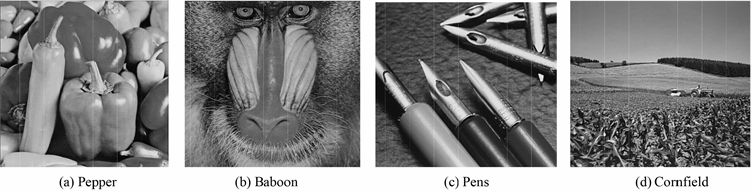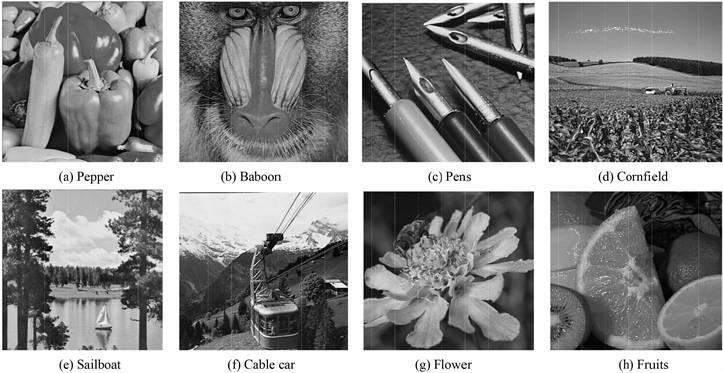基于秘密分享和直方图平移的多图像水印算法Multi-Image Watermarking Algorithm Based on Secret Sharing and Histogram Shifting

• 全文下载: PDF(8294KB)    PP.83-89   DOI: 10.12677/JISP.2019.82012
• 下载量: 71  浏览量: 114   国家自然科学基金支持

To solve the problems of small embedding capacity and low security of image watermarking algo-rithm, a watermarking image can be decomposed into multiple shadow images with the secret sharing algorithm, and then the shadow images can be embedded into multiple carrier images by histogram shifting. This paper proposes a multi-image watermarking algorithm based on secret sharing and histogram shifting. In this algorithm, the watermarking image cannot be extracted by only one shadow image, and other shadow images must be used. Therefore, the algorithm security is improved. Meanwhile, the embedding capacity of image watermarking can be enlarged by in-creasing the number of carrier images. Algorithm analyses and experimental results show that the proposed algorithm is feasible.

1. 引言

2. 理论基础

2.1. 秘密分享方案

Shamir的 $\left(k,n\right)$ 门限秘密分享方案的基本思想是：首先，要把秘密S分割成 $n$ 份影子信息；其次，分别将 $n$ 份影子信息分发给 $n$ 个参与者进行保管；最后，任意 $k\left(k\le n\right)$ 份影子信息联合起来才能恢复出S。

$f\left(x\right)={m}_{0}+{m}_{1}x+{m}_{2}{x}^{2}+\cdots +{m}_{k-1}{x}^{k-1}$(1)

2.2. 直方图平移算法

Ni等人提出了一种基于直方图平移的可逆水印算法  ，其算法思想为：首先，绘出图像的灰度直方图，并找出其中的最大值点a和最小值点b，为方便讨论，不妨设 $a ；其次，将图像中所有在 $\left[a+1,b-1\right]$ 的灰度值加1，即将 $\left[a+1,b-1\right]$ 的直方图向右平移一位，从而像素值为 $a+1$ 的像素点的个数变为0；再次，在嵌入水印时，若水印值为1，则像素值为a的灰度值加1，即为 $a+1$ ；若水印值为0，则保持 $a$ 的像素值不变；最后，提取水印信息时，顺序扫描图像，若像素灰度值为a，则提取的水印信息为0；若像素灰度值为 $a+1$，则提取的水印信息为1  。若想恢复出原始宿主图像，只需把灰度值处在区间 $\left[a+1,b\right]$ 的灰度值减1即可。

3. 新多图像水印算法

3.1. 生成影子图像

3.2. 嵌入影子图像

$k\left(k\le n\right)$ 幅载体图像为 ${I}_{\text{1}},{I}_{\text{2}},\cdots ,{I}_{k}$，从 $n$ 幅影子图像中任选 $k$ 幅即 ${W}^{1},{W}^{2},\cdots ,{W}^{k}$ 作为水印信息，分别嵌入到 $k$ 幅载体图像中。根据秘密分享方案， $k$ 幅影子图像可以恢复出原水印图像。提出的多图像水印算法嵌入影子图像的详细步骤如下：

3.3. 提取影子图像及恢复载体图像

$\begin{array}{l}{w}_{ij}^{1}\left({x}_{1}\right)={m}_{0}+{m}_{1}{x}_{1}+{m}_{2}{x}_{1}^{2}+\cdots +{m}_{k-1}{x}_{1}^{k-1}\mathrm{mod}255\\ {w}_{ij}^{2}\left({x}_{2}\right)={m}_{0}+{m}_{1}{x}_{2}+{m}_{2}{x}_{2}^{2}+\cdots +{m}_{k-1}{x}_{2}^{k-1}\mathrm{mod}255\\ \text{ }\text{ }\text{ }\text{ }\text{ }\text{ }\text{ }\text{ }\text{ }\text{ }\text{ }\text{ }\text{ }\text{ }\text{ }\text{ }\text{ }\text{ }\text{ }\text{ }\text{ }\text{ }\text{ }\text{ }\text{ }\text{ }\text{ }\text{ }\text{ }\text{ }\text{ }\text{ }\text{ }\text{ }\text{ }\text{ }\text{ }\text{ }\text{ }\text{ }\text{ }\text{ }\text{ }\text{ }\text{ }\text{ }\text{ }\text{ }\text{ }\text{ }\text{ }\text{ }\text{ }\text{ }\text{ }\text{ }\text{ }\text{ }\text{ }\text{ }\text{ }\text{ }\text{ }\text{ }\text{ }\text{ }\text{ }\text{ }\text{ }\text{ }\text{ }\text{ }\text{ }\text{ }\text{ }\text{ }\text{ }\text{ }\text{ }\text{ }\text{ }\text{ }\text{ }\text{ }\text{ }\text{ }\text{ }\text{ }\text{ }\text{ }\text{ }\text{ }\text{ }\text{ }\text{ }\text{ }\text{ }\text{ }\text{ }\text{ }\text{ }\text{ }\text{ }\text{ }\text{ }\text{ }\text{ }\text{ }\text{ }\text{ }\text{ }\text{ }\text{ }\text{ }\text{ }⋮\text{ }\text{ }\text{ }\text{ }\text{ }\text{ }\text{ }\text{ }\text{ }\text{ }\\ {w}_{ij}^{p}\left({x}_{k}\right)={m}_{0}+{m}_{1}{x}_{k}+{m}_{2}{x}_{k}^{2}+\cdots +{m}_{k-1}{x}_{k}^{k-1}\mathrm{mod}255\end{array}$(2)

4. 实验验证Figure 2. Carrier imagesFigure 3. Watermarked imagesFigure 4. Extracted watermark image

5. 算法分析

5.1. 透明性

$\text{PSNR}=10\mathrm{lg}\frac{{255}^{2}}{\frac{1}{m×n}\underset{i=0}{\overset{m-1}{\sum }}\underset{j=0}{\overset{n-1}{\sum }}{\left(X\left(i,j\right)-{X}_{W}\left(i,j\right)\right)}^{2}}$ (3)

5.2. 鲁棒性

$\text{NC}=\frac{\underset{i=1}{\overset{m}{\sum }}\underset{j=1}{\overset{n}{\sum }}W\left(i,j\right){W}^{\prime }\left(i,j\right)}{\underset{i=1}{\overset{m}{\sum }}\underset{j=1}{\overset{n}{\sum }}{W}^{2}\left(i,j\right)}$ (4)

5.3. 安全性

5.4. 嵌入容量

5.5. 算法执行效率

$T\left(n\right)=O\left(\left(n-1\right)+\text{size}+\underset{i\in U\left(\text{peak}_\text{point},\text{zero}_\text{point}\right)}{\sum }h\left(i\right)+\frac{1}{2}h\left(\text{peak}_\text{point}\right)\right)$ (5)

6. 结论

NOTES

*通讯作者。

  张景中, 陈亮, 滕鹏国, 王晓京. 一种基于阵列码的图像秘密分享方法[J]. 四川大学学报(工程科学版), 2016, 48(6): 140-148.  Ding, H.Y., Li, Z.C. and Bi, W. (2018) (k,n) Halftone Visual Cryptography Based on Shamir’s Secret Sharing. The Journal of China Universities of Posts and Telecommunications, 25, 60-76.  吴万琴, 阮文惠, 贺元香. 一种基于直方图平移和局部复杂度的可逆水印算法[J]. 南京师大学报(自然科学版), 2016, 39(3): 33-39.  于爽, 李健. 基于直方图平移的鲁棒可逆信息隐藏方案[J]. 武汉大学学报(工学版), 2018, 51(3): 268-275+282.  孙鹤鹏, 张晓强. 基于DNA编码的多图像加密算法[J]. 计算机工程与设计, 2018, 39(10): 3050-3054+3099.  Bao, L., Yi, S. and Zhou, Y.C. (2017) Combination of Sharing Matrix and Image Encryption for Lossless (k,n)-Secret Image Sharing. IEEE Transactions on Image Processing, 26, 5618-5631. https://doi.org/10.1109/tip.2017.2738561  Ding, H.Y., Li, Z.C. and Bi, W. (2018) (k,n) Halftone Visual Cryptography Based on Shamir’s Secret Sharing. The Journal of China Universities of Posts and Telecommunications, 25, 60-76.  Ni, Z.C., Shi, Y.Q., Ansari, N. and Su, W. (2006) Reversible Data Hiding. IEEE Transactions on Cir-cuits and Systems for Video Technology, 16, 354-362. https://doi.org/10.1109/TCSVT.2006.869964  李雪景, 李江隐, 康宝生. 基于图像插值和直方图平移的可逆水印算法[J]. 计算机应用研究, 2016, 33(4): 1159-1163.  罗昊, 谢晓尧, 彭长根. 基于直方图平移的加密域可逆水印算法[J]. 郑州大学学报(理学版), 2018, 50(2): 29-34.  谭亦夫, 李子臣. 基于Shamir门限秘密分享的图像可视加密算法[J]. 网络与信息安全学报, 2018, 4(7): 69-76.  Li, M., Yu, J. and Hao, R. (2017) A Cellular Automata Based Verifiable Multi-Secret Sharing Scheme without a Trusted Dealer. Chinese Journal of Electronics, 26, 313-318. https://doi.org/10.1049/cje.2017.01.026  张晓强, 王蒙蒙, 朱贵良. 图像水印算法研究新进展[J]. 计算机工程与科学, 2012, 34(4): 17-22.  王俊祥, 杨波. 基于直方图平移可逆水印的性能估计[J]. 计算机应用, 2010, 30(12): 3246-3251.